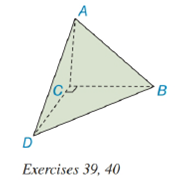Chapter 9.2, Problem 39E### Elementary Geometry for College St...

6th Edition
Daniel C. Alexander + 1 other
ISBN: 9781285195698

#### Solutions

Chapter
Section### Elementary Geometry for College St...

6th Edition
Daniel C. Alexander + 1 other
ISBN: 9781285195698
Textbook Problem
1 views

# Exercise 39 and 40 are based upon the “uniqueness of volume.”A tetrahedron (not rectangular) has vertices at A , B , C , and D . The length of the altitude from A to the base ( △ B C D ) measures 6 i n . It is given that m ∠ B C D = 90 ° , B C = 4 i n . , and C D = 8 i n . a) Find the volume of the pyramid.b) Find the length of the altitude from vertex D to the base ( △ A B C ); note that A A B C = 12 i n 2 .To determine

(a)

To find:

The volume of the pyramid.

Explanation

Given:

A tetrahedron (not rectangular) has vertices at A, B, C, and D. The length of the altitude from A to the base (BCD) measures 6in. It is given that mBCD=90°, BC=4in., and CD=8in. as below,

Properties Used:

The volume V of a pyramid having a base area B and an altitude of length h is given by V=13Bh.

Area of a right-angled triangle is 12×base×height.

Approach Used:

i) Calculate the area of the base triangle.

ii) Substitute the values of area B and the height h in equation V=13Bh to find the value of volume V.

Calculation:

From the given figure

the altitude is h=6in. Considering the base triangle BCD, having mBCD=90°, BC=4in

To determine

(b)

To find:

The length of the altitude from vertex D to the base (ABC).

### Still sussing out bartleby?

Check out a sample textbook solution.

See a sample solution

#### The Solution to Your Study Problems

Bartleby provides explanations to thousands of textbook problems written by our experts, many with advanced degrees!

Get Started

#### In Exercises 2528. solve the equation by factoring. 28. 2x4 + x2 = 1

Applied Calculus for the Managerial, Life, and Social Sciences: A Brief Approach

#### Differentiate the function. j(x) = x2.4 + e2.4

Single Variable Calculus: Early Transcendentals, Volume I

#### True or False: converges if and only if .

Study Guide for Stewart's Multivariable Calculus, 8th

#### True or False: n=1(1)nnn+3 converges.

Study Guide for Stewart's Single Variable Calculus: Early Transcendentals, 8th